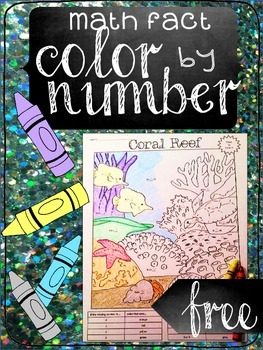# Math Fact Color-by-Number Freebie (addition/subtraction)1st - 2nd
Standards
Resource Type
Formats Included
• PDF
Pages
1 page

### Description

A fun way to practice math facts! Students solve the equations on the picture and the answer they get corresponds with the color to use in that area.

To align with Common Core the unknowns are in all positions (ex: 4+___=9)

You can find the whole set of 8 color by numbers here:
**Math Fact Color By Numbers

More Common Core math resources:
**Attributes of Shapes
**Math Fact Running Record
**Open Number Line Practice Pack

Total Pages
1 page
N/A
Teaching Duration
30 minutes
Report this Resource to TpT
Reported resources will be reviewed by our team. Report this resource to let us know if this resource violates TpT’s content guidelines.

### Standards

to see state-specific standards (only available in the US).
Use addition and subtraction within 20 to solve word problems involving situations of adding to, taking from, putting together, taking apart, and comparing, with unknowns in all positions, e.g., by using objects, drawings, and equations with a symbol for the unknown number to represent the problem.
Add and subtract within 20, demonstrating fluency for addition and subtraction within 10. Use strategies such as counting on; making ten (e.g., 8 + 6 = 8 + 2 + 4 = 10 + 4 = 14); decomposing a number leading to a ten (e.g., 13 - 4 = 13 - 3 - 1 = 10 - 1 = 9); using the relationship between addition and subtraction (e.g., knowing that 8 + 4 = 12, one knows 12 - 8 = 4); and creating equivalent but easier or known sums (e.g., adding 6 + 7 by creating the known equivalent 6 + 6 + 1 = 12 + 1 = 13).
Fluently add and subtract within 20 using mental strategies. By end of Grade 2, know from memory all sums of two one-digit numbers.